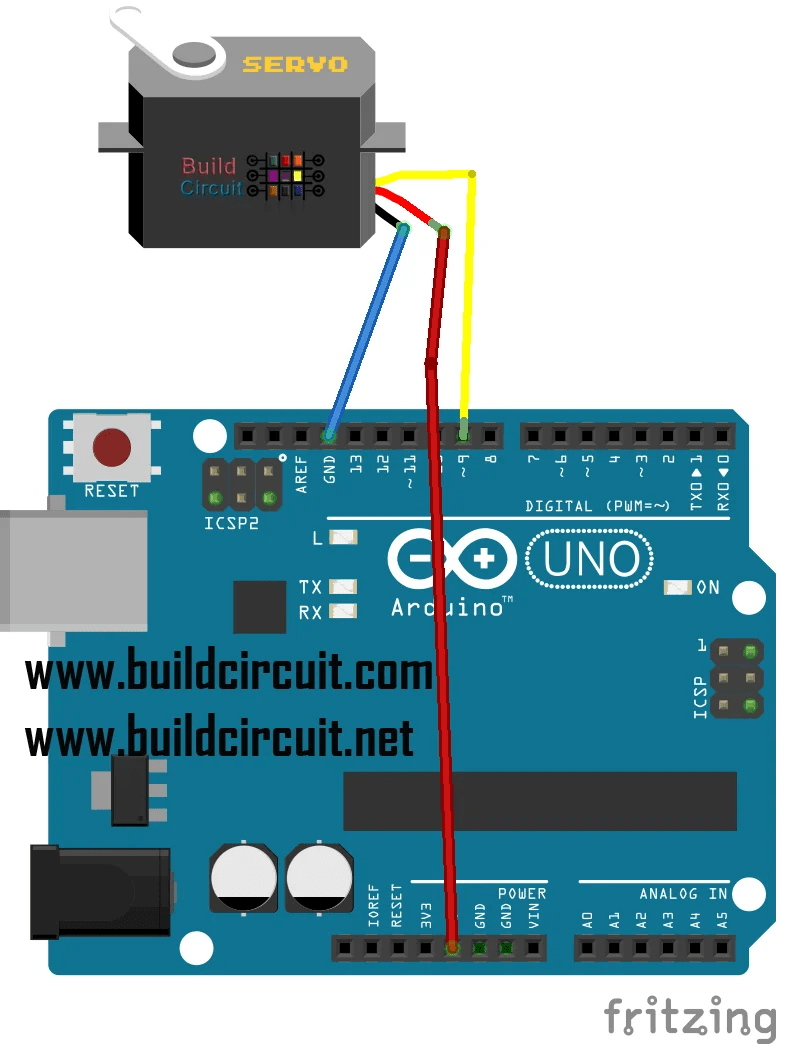# Arduino Project 5- How to use a servo with Arduino

In this tutorial, we will learn how to use a servo motor.

A Servo is a motor capable of making maximum rotations of 270° and is widely used in robotics and modeling.

It requires a library (<Servo.h>) that is already installed on the Arduino IDE software.

In this tutorial, we will vary the position of the servo every half second, you can vary the pause time for each rotation by changing the time (in milliseconds) in the delay brackets (500). In our case, the delay is 500 ms which means that the servo after having turned to a certain degree/angle pauses for half a second.

List of components:

Name Quantity Component
U1 1 Arduino Uno R3
M1 1 Servo